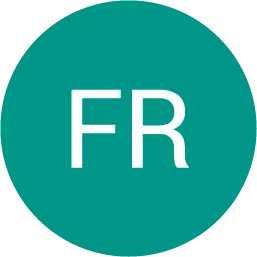, 23.06.2019 08:30 deanlmartin

Ben uses a compass and a straightedge to bisect angle pqr, as shown below: the figure shows two rays qp and qr having a common end point q. an arc drawn from q cuts the ray qp at a and the ray qr at b. two small arcs intersect between the lines qp and qr. the point of intersection of the arcs is labeled s. the points q, s, and t are joined with a line. a. ∠aqs ≅ ∠bqs when lines qp and qr are equal. b. ∠aqs ≅ ∠bqs when segments aq and bq are equal. c. ∠aqs ≅ ∠bqs when angle asq is equal to angle bqs. d. ∠aqs ≅ ∠bqs when angle aqs is equal to angle bsq.Another question on MathematicsMathematics, 21.06.2019 17:00
The volume of a cube is 8 in to the 3rd power. how long is each side?Mathematics, 21.06.2019 17:30
What term makes it inconsistent y=2x - 4 ?Mathematics, 21.06.2019 18:30
Which of the following is the result of expandingMathematics, 21.06.2019 18:50
In which form is the following function written? y = - 2(x – 3)(x + 5)
Ben uses a compass and a straightedge to bisect angle pqr, as shown below: the figure shows two ray...
QuestionsQuestions on the website: 14524237